# C Pointers

Pointers are very important in C language. The correct understanding and use of pointers is important to successful C programming. There are the following reasons behind this:

• pointers provides the means by which function can modify their calling arguments
• pointers supports dynamic allocation
• pointers can improve the efficiency of certain routines.
• pointers provides support for dynamic data structures, such as binary trees and linked lists

## What are Pointers ?

A pointer is a variable, holds a memory address. And this address is the location of another object (generally another variable) in memory. For instance, if one variable contains the address of another variable, then the first variable is said to point to the second. Here is the figure for illustration of this situation: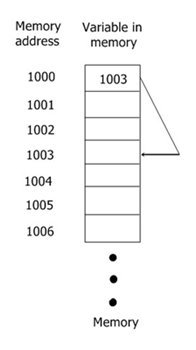## Pointer Variables

If a variable is going to be a pointer, it must be declared like this:

`type *name;`

As you can see, a pointer declaration consists of a base type, an *, and then the variable name. Here type is the base type of the pointer and may be any valid type. And the name of the pointer variable is specified by name.

The base type of the pointer defines the type of object to which the pointer will point.

## Pointer Operators

There are the following two pointer operators:

1. *
2. &

The & is a unary operator, returns the memory address of its operand.

Always remember that a unary operator only requires one operand. Here is the example.

`a = &count;`

this places into m, the memory address of the variable count. This address is the computer's internal location of the variable.

The pointer *, is the complement of &. It is a unary operator that returns the value located at address which follows. It is also called as 'value at address' operator. For instance, if m contains the memory address of the variable count, then

`n = *m;`

places the value of count into n. That is, if count have the value 10, then n will also having the same value which is 10.

## Pointer Assignments

Here is the example program of pointer assignments:

```/* C Pointers - Pointer Assignments
* This program illustrates the
* pointer assignments in C
*/

#include<stdio.h>
#include<conio.h>
void main()
{
int a = 99;
int *ptr1, *ptr2;
clrscr();

ptr1 = &a;
ptr2 = ptr1;

// printing the value of a twice
printf("Value of ptr1 : %d\n", *ptr1);
printf("Value of ptr2 : %d\n\n", *ptr2);

// printing the address of a twice
printf("Address pointed to by ptr1 : %p\n", ptr1);
printf("Address pointed to by ptr2 : %p\n\n", ptr2);

getch();
}```

In this program, after this assignment sequence:

```ptr1 = &a;
ptr2 = ptr1;```

ptr1 and ptr2 both point to a. Here is the sample output of this C program: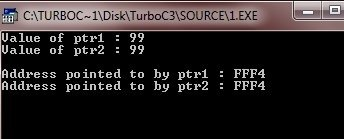Note - Always keep in mind that the address are displayed by using the %p printf() format specifier, that causes the printf() to display an address in the format used by the host computer.

## Pointer Conversion

Pointer conversions required when pointer of one type is going to point other data type's variable or going to assign other type's pointer.

Here is the program which attempts to assign the value of a and b, through the pointer ptr. This program compiles without an error, but the output will not the desired output.

```/* C Pointer - Pointer Conversion
* This program illustrates the
* pointer conversion in C
*/

#include<stdio.h>
#include<conio.h>
void main()
{
double a = 100.1, b;
int *ptr;
clrscr();

/* here this statement causes ptr (which
* is an integer pointer) to point to a
* double.
*/
ptr = (int *) &a;

/* and here this statement doesn't operate
* as expected.
*/
b = *ptr;  // this attempt to assign b the value of a through ptr

// and now this statement won't output as 100.1
printf("The (incorrect) value of a is : %f", b);

getch();
}```

Here is the output of this C program: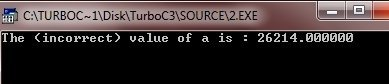## Generic Pointer

In C language, it is permissible to assign a void * pointer to any other type of pointer. It is also permissible to assign any other type of pointer to a void * pointer. A void * pointer is called a generic pointer. The void * pointer is used to specify a pointer whose base type is unknown. The void * type allows a function to specify a parameter that is capable of receiving any type of pointer argument without reporting a type mismatch.

## Pointer Arithmetic

In C language, there are only the following two arithmetic operations that you can use on pointers:

• subtraction

To understand about the pointer arithmetic pointer, let's assume that a pointer ptr1 is an integer pointer with a current value 1000. Also, assume that ints are 2 bytes long. So after the expression

`ptr++;`

ptr contains 1002. The reason for this is that each time when ptr is incremented, it will point to the next integer. The same concept is for decrements.

Now concentrate on this example program:

```/* C Pointer - Pointer Arithmetic
* This program illustrates the
* concept of pointer arithmetic
*/

#include<stdio.h>
#include<conio.h>
void main()
{
int *ptri, *ptrd, i;
clrscr();

ptri = ptrd = 1000;

printf("Incrementing Integer Pointer...\n");
for(i=0; i<5; i++)
{
printf("%d\n", ptri);
ptri++;
}
printf("\n");
printf("Decrementing Integer Pointer...\n");
for(i=0; i<5; i++)
{
printf("%d\n", ptrd);
ptrd--;
}

getch();
}```

Here is the sample run of this C program: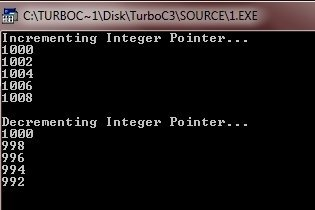## Pointer Comparisons

In C language, you can also compare two pointers in a relational expression. For instance, here given two pointers ptr1, and ptr2, this statement is totally valid in C:

```if(ptr1 < ptr2)
{
printf("ptr1 points to lower memory than ptr2");
}```

Here is a simple example program to illustrates the concept of pointer comparisons. Just concentrate on this, this program is based on the stack. To stop the program, just enter -1.

```/* C Pointer - Pointer Comparisons
* This program illustrates the
* concept of pointer comparisons
* in C language
*/

#include<stdio.h>
#include<conio.h>
#include<stdlib.h>

#define SIZE 50

void push(int);
int pop(void);
int *tos, *ptr;
int stack[SIZE];

void main()
{
int val;
clrscr();

tos = stack;   // now tos points to the top of the stack
ptr = stack;   // initialize ptr

do
{
printf("Enter value : ");
scanf("%d", &val);
if(val != 0)
{
push(val);
}
else
{
printf("value on top is %d\n", pop());
}
}while(val != -1);

getch();
}
void push(int i)
{
ptr++;
if(ptr == (tos+SIZE))
{
printf("Stack Overflow..!!\n");
exit(1);
}
*ptr = i;
}
int pop(void)
{
if(ptr == tos)
{
printf("Stack Underflow..!!\n");
exit(1);
}
ptr--;
return *(ptr+1);
}```

Here is the sample run of this program: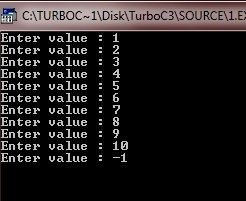## Pointers and Arrays

In C language, there is a close relationship between pointers and arrays. Let's consider this code fragment:

```int arr, *ptr;
ptr = arr;```

Here, ptr has been set to the address of the first array element in arr. And to access any element from the array like to access fourth element in arr, you could write this code fragment:

`arr;`

Or

`*(ptr+3);`

Here is the example program. Just concentrate on this program.

```/* C Pointer - Pointers and Arrays */

#include<stdio.h>
#include<conio.h>
void main()
{
int arr = {1, 2, 3, 4, 5, 6, 7, 8, 9, 10};
int *ptr;
int i;
clrscr();

ptr = arr;
for(i=0; i<10; i++)
{
printf("arr[%d] = %d\t *(ptr+%d) = %d\n", i, arr[i], i, *(ptr+i));
}

getch();
}```

Here is the sample run of this C program: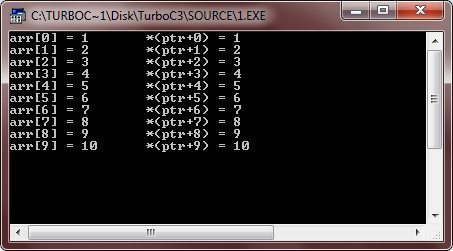## Multiple Indirection

In C language, you can also have a pointer point to another pointer that points to the target value. This situation is called multiple indirection, or pointers to pointers. Here this figure illustrates the concepts of multiple indirection: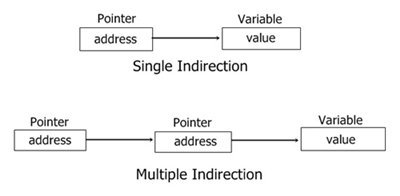Now let's take a example of multiple indirection.

## C Pointer Multiple Indirection Example

To access the target value indirectly pointed to by a pointer to a pointer, then you must apply the asterisk operator twice as shown here in the example program:

```/* C Pointer - Multiple Indirection
* This program illustrates the concepts
* of C Pointer Multiple Indirection or
* C pointers to pointers
*/

#include<stdio.h>
#include<conio.h>
void main()
{
int a;
int *ptrs, **ptrm;
clrscr();

a = 10;
ptrs = &a;
ptrm = &ptrs;

printf("Value = %d", **ptrm);   // this prints the value of a

getch();
}```

Here is the sample output of this C program: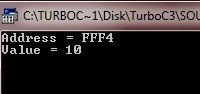## Pointer Initialization

In C language, after a non-static, local pointers is declared but before it has been assigned a value, it contains an unknown value. Global and static local pointers are automatically initialized to null.

Important - Should you try to use the pointer before giving it a valid value, you will crash your program and may be your computer's operating system as well, a very nasty type of error!.

Now concentrate on this example program to understand the concept of pointer initialization in C language.

```/* C Pointer - This program illustrates the
* concept of pointer initialization in C
*/

#include<stdio.h>
#include<conio.h>
#include<string.h>

int search(char *p[], char *name);

char *names[] = {"programmer", "learner", "student", "coder",
"decoder", "teacher", "professor", "enginner",
"developer", NULL};  // null pointer constant ends the list

void main()
{
char str;
clrscr();

printf("Who are you ? ");
scanf("%s", str);
if(search(names, str) != -1)
{
printf("%s is in the list.\n", str);
}
else
{
printf("%s is not in the list.\n", str);
}

getch();
}

int search(char *p[], char *name)
{
register int i;
for(i=0; p[i]; i++)
{
if(!strcmp(p[i], name))
{
return i;
}
}
}```

Here is the four sample run of this program, two for found, and other two for not found.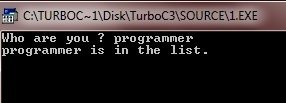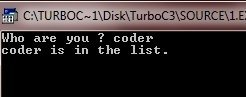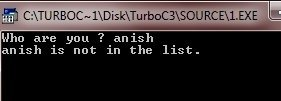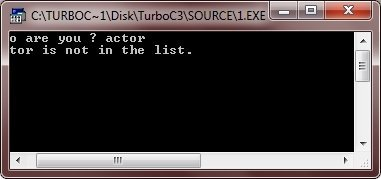## Pointers to Functions

A particularly confusing but powerful feature of C is function pointer. As you know, a function has a physical location in memory that can be assigned to a pointer. This address is the entry point of the function and it is the address used when the function is called. Once a pointer points to a function, the function can be called through that pointer. And an important things is that, you can also allow functions to be passed as arguments to other functions. Here is the example program.

```/* C Pointer - Pinters to Functions */

#include<stdio.h>
#include<conio.h>
#include<string.h>

void check(char *a, char *b, int (*cmp)(const char *, const char *));

void main()
{
char str1, str2;
int (*ptr)(const char *, const char *);   // this is a function pointer
clrscr();

ptr = strcmp;    // assign address of strcmp to p
printf("Enter any two strings :\n");
gets(str1);
gets(str2);

check(str1, str2, ptr);    // pass address of strcmp via p

getch();
}
void check(char *a, char *b, int (*cmp)(const char *, const char *))
{
printf("\n");
if(!(*cmp)(a, b))
{
printf("Both the strings are equal");
}
else
{
printf("Both the strings are not equal");
}
}```

Here is the two sample output of this C program, one for equal and other for not equal: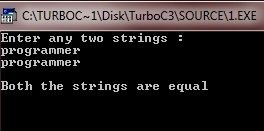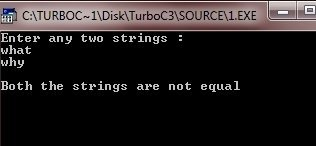In the above C program, examine this declaration for p in main() function.

`int (*ptr)(const char *, const char *);`

This declaration tells the compiler that ptr is a pointer to a function that has two const char * parameters, and returns an int result. The parentheses around p are necessary in order for the compiler to properly interpret this declaration. You must have to use the similar form when declaring other function pointers, although the return type and the parameters of the function may differ.

Let's take a look at one more example to understand the concept of pointers to functions completely.

```/* C Pointer - Pinters to Functions */

#include<stdio.h>
#include<conio.h>
#include<ctype.h>
#include<stdlib.h>
#include<string.h>

void check(char *a, char *b, int (*cmp)(const char *, const char *));
int compval(const char *a, const char *b);

void main()
{
char str1, str2;
clrscr();

printf("Enter any two values or two strings :\n");
gets(str1);
gets(str2);

if(isdigit(*str1))
{
printf("Testing values for equality.\n");
check(str1, str2, compval);
}
else
{
printf("Testing strings for equality.\n");
check(str1, str2, strcmp);
}
getch();
}
void check(char *a, char *b, int (*cmp)(const char *, const char *))
{
printf("\n");
if(!(*cmp)(a, b))
{
printf("Equal");
}
else
{
printf("Not equal");
}
}
int compval(const char *a, const char *b)
{
if(atoi(a) == atoi(b))
{
return 0;
}
else
{
return 1;
}
}```

Here is the 5 sample output of this program, two for string's equal and not equal, and other three for value's equal and not equal.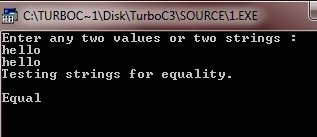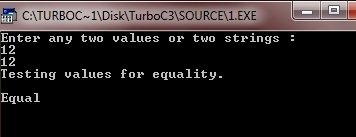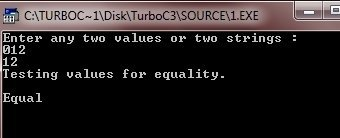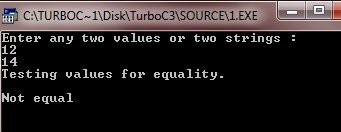As you can see, from the above output (run 4), the values 012 and 12 are equal.

Tools
Calculator